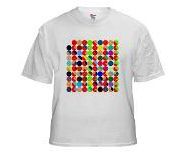### Make your own 'prime factorization' diagram

The Prime Factorization Sweater is a lovely idea and I thought it would be fun to reproduce the same idea electronically so that I could print out a poster version for home.

Enter Processing.

With it I've developed a small program that produces a diagram of the first 100 numbers and for each number there's a circle broken up into arcs.  Each arc is a prime factor.  As in the original sweater each factor gets a unique color (assigning unique colors is rather complex and I ended up using the color difference method based on CMC l:c and a nice online tool that does the work for you).

Here's the finished product.  The top left corner is the number 1 and the numbers read right to left.  So the first red circle is a prime number (2), the second the next number (3, which is prime) and so on.

There's also an option to print the numbers involved.

The source code is in the pfd repository on GitHub and licensed under GPLv2. Processing is a really nice environment for this sort of rapid hacking of anything graphical. See, for example, how I used it to visualize Ikea Lillabo Train Set layouts.

PS After encouragement in the comments from the person who had the original idea for the prime factorization sweater I've made a CafePress store in which you can buy men's and women's T-shirts printed with the prime factorization diagram.Sondy said…
WOW! Since I was knitting, I never once thought about using circles! I totally love this. Now put it on a t-shirt!Sondy said…
WOW! I totally love this! Since I was knitting, I never once thought about using circles. For printing the charts, this is totally cool! (Think about making a t-shirt!)Unknown said…
Nice. One small point, the numbers read right to left is the wrong way around.This is the best idea about integer factorization, written here is to let more people know and participate.
A New Way of the integer factorization
1+2+3+4+……+k=Ny,(k<N/2),"k" and "y" are unknown integer,"N" is known Large integer.
True gold fears fire, you can test 1+2+3+...+k=Ny(k<N/2).
How do I know "k" and "y"?
"P" is a factor of "N",GCD(k,N)=P.

Two Special Presentation:
N=5287
1+2+3+...k=Ny
Using the dichotomy
1+2+3+...k=Nrm
"r" are parameter(1;1.25;1.5;1.75;2;2.25;2.5;2.75;3;3.25;3.5;3.75)
"m" is Square
(K^2+k)/(2*4)=5287*1.75 k=271.5629(Error)
(K^2+k)/(2*16)=5287*1.75 k=543.6252(Error)
(K^2+k)/(2*64)=5287*1.75 k=1087.7500(Error)
(K^2+k)/(2*256)=5287*1.75 k=2176(OK)
K=2176,y=448
GCD(2176,5287)=17
5287=17*311

N=13717421
1+2+3+...+k=13717421y
K=4689099,y=801450
GCD(4689099,13717421)=3803
13717421=3803*3607

The idea may be a more simple way faster than Fermat's factorization method(x^2-N=y^2)!
True gold fears fire, you can test 1+2+3+...+k=Ny(k<N/2).
More details of the process in my G+ and BLOG.

Email:[email protected]Unknown said…
Great job, John! I like it a lot:)

One simple modification would really make the primes visually easy to identify: reduce the radius, maybe even by as much as 1/2.

Again, nice visualization. I will be sharing it with my weekly math/philosophy group, the Wing Circle, as the meeting topic is "Prime Numbers". Feel free to check out our facebook page here http://tinyurl.com/WC-EventHistoryList.

Sincerely,

swami_mathtravelerDaniel said…
This has gained a lot of interest on G+ through a post by Richard Green:

I have really gotten a kick out of it and made a few modifications to the code to change the modulus with a single setting. I submitted a pull request on GitHub for you to review.

Thanks for creating something so cool!